+
Rationalizing the Denominator
Solving Linear Equations and Inequalities
0
of 0 possible points

# Rationalizing the Denominator

Author: Sophia Tutorial
##### Description:

Simplify algebraic fractions with a radical in the denominator.

(more)

Sophia’s self-paced online courses are a great way to save time and money as you earn credits eligible for transfer to many different colleges and universities.*

No credit card required

46 Sophia partners guarantee credit transfer.

299 Institutions have accepted or given pre-approval for credit transfer.

* The American Council on Education's College Credit Recommendation Service (ACE Credit®) has evaluated and recommended college credit for 33 of Sophia’s online courses. Many different colleges and universities consider ACE CREDIT recommendations in determining the applicability to their course and degree programs.

Tutorial
what's covered
1. Rationalizing the Denominator

# 1. Rationalizing the Denominator

It is considered bad practice to have a radical in the denominator of a fraction. When this happens we multiply the numerator and denominator by the same thing in order to clear the radical. In the lesson on dividing radicals we talked about how this was done with monomials. Here we will look at how this is done with binomials.

If the binomial is in the numerator the process to rationalize the denominator is essentially the same as with monomials The only difference is we will have to distribute in the numerator.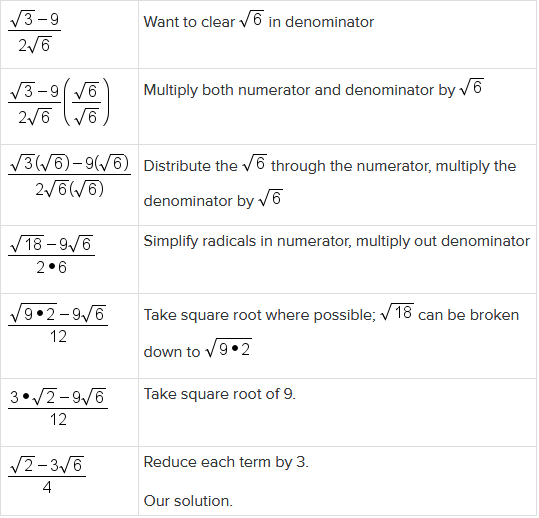hint
It is important to remember that when reducing the fraction we cannot reduce with just the 3 and 12 or just the 9 and 12. When we have addition or subtraction in the numerator or denominator we must divide all terms by the same number. As we are rationalizing it will always be important to constantly check our problem to see if it can be simplified more. We ask ourselves, can the fraction be reduced? Can the radicals be simplified? These steps may happen several times on our way to the solution.

If the binomial occurs in the denominator we will have to use a different strategy to clear the radical. Consider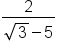, if we were to multiply the denominator by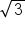we would have to distribute it and we would end up with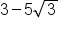. We have not cleared the radical, only moved it to another part of the denominator. So our current method will not work. Instead we will use what is called a conjugate. A conjugate is made up of the same terms, with the opposite sign in the middle. So for our example with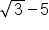in the denominator, the conjugate would be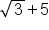term to know
Conjugate
The conjugate of a binomial is a binomial with the opposite sign between its terms.

The advantage of a conjugate is when we multiply them together we have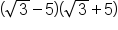, which is a difference and a sum. We know when we multiply these, we get a difference of squares. Squaringand 5, with subtraction in the middle gives the product: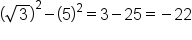Our answer when multiplying conjugates will no longer have a square root, which is exactly what we want.

big idea
To rationalize a denominator containing a radical expression, multiply the fraction using its conjugate. The product will no longer contain a radical.

EXAMPLE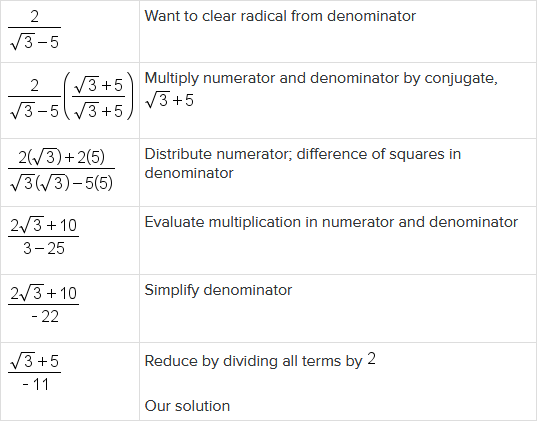In the previous example, we could have reduced by dividing by -2 instead of 2, giving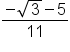. Both answers are correct.

EXAMPLE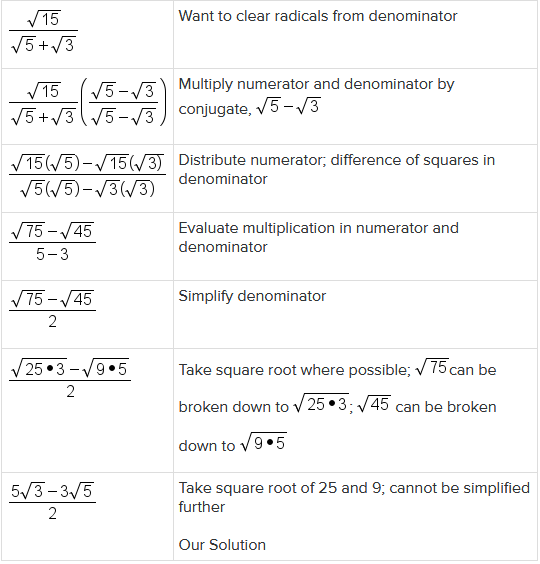The same process can be used when there is a binomial in the numerator and denominator. We just need to remember to FOIL out the numerator.

EXAMPLE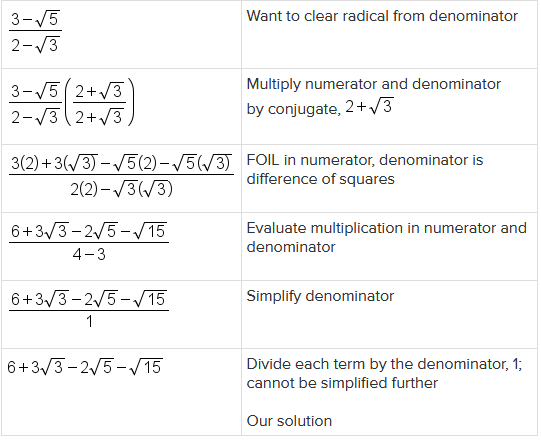EXAMPLE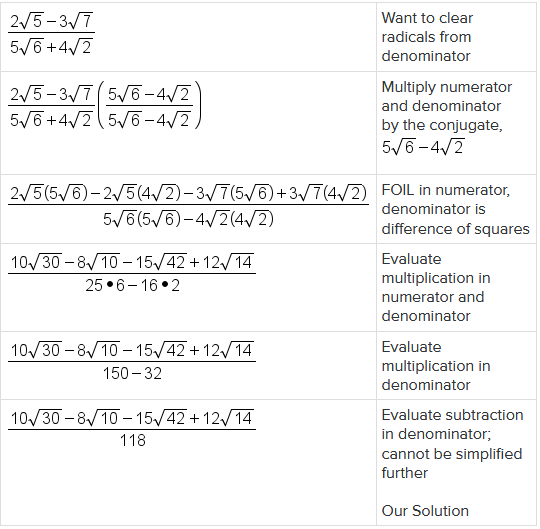did you know
During the 5th century BC in India, Aryabhata published a treatise on astronomy. His work included a method for finding the square root of numbers that have many digits.

summary
Rationalizing the denominator involves multiplying by a conjugate in both the denominator and the numerator of a fraction and then simplifying. The reason that we do that is because having an irrational radical in the denominator of a fraction is not considered simplified. The conjugate of the √a + b is just √a - b. We just use the opposite sign in between the two terms. The conjugate of just a radical by itself is that same radical.

Source: Adapted from "Beginning and Intermediate Algebra" by Tyler Wallace, an open source textbook available at: http://wallace.ccfaculty.org/book/book.html

Terms to Know
Conjugate

The conjugate of a binomial is a binomial with the opposite sign between its terms.

Rating